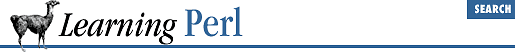home | O'Reilly's CD bookshelfs | FreeBSD | Linux | Cisco | Cisco Exam

### 3.7 Variable Interpolation of Arrays

Like scalars, array values may be interpolated into a double-quoted string. A single element of an array will be replaced by its value, like so:

```@fred = ("hello","dolly");
\$y = 2;
\$x = "This is \$fred's place";     # "This is dolly's place"
\$x = "This is \$fred[\$y-1]'s place";  # same thing```

Note that the index expression is evaluated as an ordinary expression, as if it were outside a string. It is not variable interpolated first.

If you want to follow a simple scalar variable reference with a literal left square bracket, you need to delimit the square bracket so it isn't considered part of the array, as follows:

```@fred = ("hello","dolly");  # give value to @fred for testing
\$fred = "right";
# we are trying to say "this is right"
\$x = "this is \$fred";    # wrong, gives "this is dolly"
\$x = "this is \${fred}";  # right (protected by braces)
\$x = "this is \$fred".""; # right (different string)
\$x = "this is \$fred\";   # right (backslash hides it)```

Similarly, a list of values from an array variable can be interpolated. The simplest interpolation is an entire array, indicated by giving the array name (including its leading ``` @``` character). In this case, the elements are interpolated in sequence with a space character between them, as in:

```@fred = ("a","bb","ccc",1,2,3);
\$all = "Now for @fred here!";
# \$all gets "Now for a bb ccc 1 2 3 here!"```

You can also select a portion of an array with a slice:

```@fred = ("a","bb","ccc",1,2,3);
\$all = "Now for @fred[2,3] here!";
# \$all gets "Now for ccc 1 here!"
\$all = "Now for @fred[@fred[4,5]] here!"; # same thing```

Once again, you can use any of the quoting mechanisms described earlier if you want to follow an array name reference with a literal left bracket rather than an indexing expression.3.6 as an Array3.8 Exercises